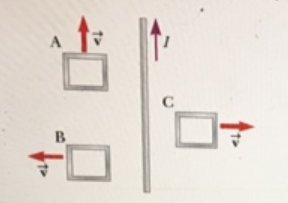# Problem: Three loops of wire move near a long straight wire carrying a current as in the following figure. (a) What is the direction of the Induced current, if any, in loop A? A) clockwise B) counterclockwiseC) No current is induced. (b) What is the direction of the induced current, if any, in loop B?A) clockwise B) counterclockwiseC) No current is Induced.(c) What is the direction of the induced current, if any, in loop C?A) clockwise B) counterclockwiseC) No current is induced.

###### FREE Expert Solution

(a)

There is no change in the magnetic flux in loop A.

Therefore, there is no induced current.

81% (370 ratings)###### Problem Details

Three loops of wire move near a long straight wire carrying a current as in the following figure.

(a) What is the direction of the Induced current, if any, in loop A?
A) clockwise
B) counterclockwise
C) No current is induced.

(b) What is the direction of the induced current, if any, in loop B?
A) clockwise
B) counterclockwise
C) No current is Induced.

(c) What is the direction of the induced current, if any, in loop C?

A) clockwise
B) counterclockwise
C) No current is induced.